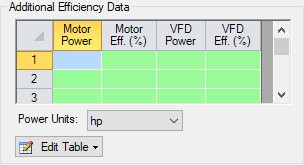### Pump Efficiency Data

Best Efficiency Point (BEP)

By defining a power or efficiency curve, the Best Efficiency Point of the pump can be found. Optionally, the calculated value can be overridden with a user-defined value in Pump Configuration. It is generally desirable to operate pumps as close to the BEP as possible. The Pump Summary can display what the BEP is, as well as how far off (in % of BEP) of BEP the pump is operating in the model.

Power and Efficiency Calculations

Various stages of power or efficiency data relating to a pump assembly can be added to a pump junction.

The most basic value that can be accounted for is the pump efficiency or power curve. This curve, entered in Pump Configuration, is used to determine the power/efficiency being delivered to the pump itself - the Shaft Power.

AFT Fathom uses the head curve of the pump with the system configuration to determine the total dynamic head (ΔH) and flow (Q) through the pump. Along with the fluid density (ρ) at the pump, the fluid power, or Ideal Power, can be directly calculated:With a pump power or efficiency curve, the missing value can be calculated with the curve value at the given flow:On a pump's optional tab, further efficiency information can be defined:Figure 1: Additional Efficiency Data

Entries in this table must come in pairs - both Power and Efficiency values must be present for a calculation to take place, but only one pair is required. That is, only Motor data or only VFD data can be entered in addition to filling out all four columns.

Motor Power in this instance refers to the output power of the motor - the Shaft Power calculated previously, delivered to the pump. Because the Shaft Power is already known, the Motor efficiency can be looked up from this table. Motor Input Power can then be calculated:VFD Power is used in a similar fashion. The VFD Power in Figure 1 refers to the Output power of the VFD, which is identical to the Motor Input power. Because Motor Input Power was calculated, VFD efficiency can be looked up in the table, and VFD Input Power calculated with:This power is considered the Overall Power, viewable in the Pump Summary along with all of the other above values. Whenever an efficiency value is left undefined, it is assumed to be 1 (perfectly efficient).